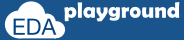# randomization in SystemVerilog

Randomization is the process of making something random; SystemVerilog randomization is the process of generating random values to a variable. Verilog has a \$random method for generating the random integer values. This is good for randomizing the variables alone, but it is hard to use in case of class object randomization. for easy randomization of class properties, SystemVerilog provides rand keyword and randomize() method.

## random variables

The class variables which get random values on randomization are called random variables. In order to make variables as random variables, Class variables need to be declared using the rand and randc type-modifier keywords.

Following types can be declared as rand and randc,
• singular variables of any integral type
• arrays
• arrays size
• object handle's

## rand keyword

Variables declared with the rand keyword are standard random variables. Their values are uniformly distributed over their range.

`rand bit [3:0] addr;`

addr is a 4-bit unsigned integer with a range of 0 to 15. on randomization this variable shall be assigned any value in the range 0 to 15 with equal probability.

## randc keyword

randc is random-cyclic. For the variables declared with the randc keyword, on randomization variable values don't repeat a random value until every possible value has been assigned.

`randc bit wr_rd;`

In order to randomize the object variables, the user needs to call randomize() method.

example:
`object.randomize();`

## randomization example

In the example below,
Two variables addr1 and addr2 of same bit type are declared as rand and randc respectively, observe the randomized values of addr1 and addr2.

addr1 - takes the random value on every randomization
addr2 - takes the random value on every randomization, but takes random value until every possible value has been assigned

```//class
class packet;
endclass

module rand_methods;
initial begin
packet pkt;
pkt = new();
```
```    repeat(10) begin
pkt.randomize();
end
end
endmodule```

Simulator Output

Execute the above code on